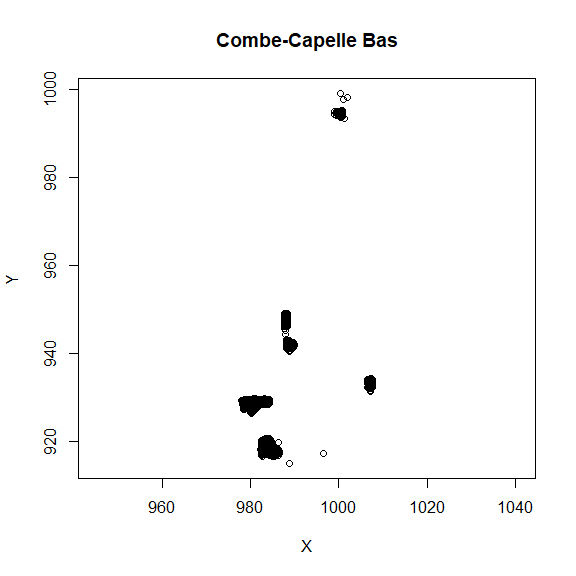The following code demonstrates how R can be used to read the Combe-Capelle Bas SQLite database for plotting and analysis.

knitr::opts_chunk$set(echo = TRUE, warning = FALSE) library(plyr) library(dplyr) library(RSQLite) library(knitr) Read the tables from the database into dataframes. Then merge the context and xyz tables together, and merge the lithics and context tables (so that Layer becomes part of the lithics table). Note that an example is not given here for the smallfinds, but the table is opened anyway here for example. con = DBI::dbConnect(RSQLite::SQLite(), dbname = "data/ccbas.sqlite") context = dbReadTable(con, "cc.context") %>% select(-unit, -idno) xyz = dbReadTable(con, 'cc.xyz') %>% mutate(squid = squid_id) %>% select(-squid_id, -unit, -id) lithics = dbReadTable(con, "cc.ccbas_lithics") %>% mutate(squid = squid_id) %>% select(-squid_id, -unit, -id, -idno) smallfinds = dbReadTable(con, "cc.ccbas_smallfinds") dbDisconnect(con) cc = join(context, xyz, by = c('squid'), type = 'right') lithics = join(lithics, context, by = c('squid'), type = 'left') Make a plot of the location of the items in the database using only Suffix = 0 points. plot(cc$x[cc$suffix == 0], cc$y[cc$suffix == 0], asp = 1, main = 'Combe-Capelle Bas', xlab = 'X', ylab = 'Y')Provide a breakdown of the basic types of lithics in the database. kable(table(Dataclass = lithics$dataclass))
Dataclass Freq
BIFACE 6
BIFFRAG 2
COMPFLAKE 3081
COMPTOOL 741
CORE 380
COREFRAG 278
DISTFLAKE 1089
DISTTOOL 210
MANUPORT 35
MEDFLAKE 1689
MEDTOOL 400
PROXFLAKE 1194
PROXTOOL 157
RETFLAKE 1
ROGNON 67
SHATTER 1035